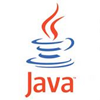# What is B-Tree?

Connect withLearn, what is BTree data structure or binary tree data structure? how to use BTree? properties of a binary tree, algorithm to insert Btree. the basic building block of BTree/binary tree data structure.

## 1. Overview of BTree Data structure

Well, you must have so many questions in your mind when you heard about anything which has more impact on the data structure or various algorithm. What is the B-tree data structure? Its widely used data structure in all the levels of the software development process.
where it can be used? How to use this BTree? B-Tree is an extension of Binary Search Tree (BST). B-Tree of order m satisfy the following properties.

BTree is used in almost all the database for indexing. Binery tree is basic building block of search algorithm, without BTree we can’t imagine the search and indexing world of software.

## 2. Properties of Binary Tree

B-Tree is an extension of Binary Search Tree (BST). B-Tree of order m satisfy the following properties.

1. Each node has at most m children `ceil(m/2)` i.e. `ceil(5/2)=3`
2. Each internal node has atmost `ceil(m/2)` children.
3. Root has at least 2 children , if it is not leaf.
4. A non-leaf node with k children has `(k-1)` keys.
5. All leaves appear in same level.

## 3. Algorithm to insert B-Tree

Let us consider , x be a number of node and m be the number of keys in a node in B-Tree data structure. Let us discus step by step.

1. Use Search procedure like m-array tree, to find leave node where x should be placed.
2. Add x to appropriate place in leave in ascending order.
3. if there are `m-1` or fewer key then done.
4. if more than `m-1` key i.e. overflow, then split the node into 2 equal parts and one middle element.
5. Add mid to parent and make these two new nodes as its child.
6. if there is a overflow in parent split it , we continue till o overflow occurs or root it self splits.

## 4. Reference

I hope you enjoyed this post of what is binary tree data structure or B-Tree data structure? and you can visit design patterns tutorial for more details.

Visit Wiki page for Binary Tree data structure.
Suggestions or comments are welcome to improve this post. cheers! Happy learning! 🙂

Connect with